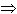SEARCH HOMEMath Central Quandaries & QueriesQuestion from Bharathi, a student: given a circle with radius r and a point x,y on its circumference,output two other points x1,y1 and x2,y2 on the circle so that all 3 points form a equilateral triangle. i want a clear explanation pleaseHi Bharathi,

I presume you have the center of the circle (h, k) as well. Here's how I would do this. The gist is to convert it to a polar co-ordinate system, rotate to find the other two points, then convert back to cartesians.

1. Apply the transformation xx - h and yy - k to slide the centre to the origin.
2. Switch to polar coordinates (r, θ) using the relationship θ = tan-1(y/x).
3. Apply two 120o angular transformations to find the two other points in polar form (r, θi).
4. Convert both new points back to the cartesian coordinates using the relationships xi = r cos(θi) and yi = r sin(θi).
5. Apply the transformation xixi + h and yiyi + k for both points to move the centre back.

Hope this helps,
Stephen La Rocque.Math Central is supported by the University of Regina and The Pacific Institute for the Mathematical Sciences.